# WordCount

1.读文件：

while((c =sr.Read()) != -1) //一个一个读取字符
{
charNumber++;  //没读一个字符，字符数+1
if ((char)c == ',' || (char)c == ' ')
wordNumber++;     //遇到逗号或者空格，单词数+1
if ((char)c == '\n') //遇到转行符，行数+1
lineNumber++;
}

2.写文件：

//type存入的是指令，分别是字符、单词和行数的标志
bool writeChar = false;  //各种指令
bool writeWord = false;
bool writeLine = false;
for(int i = 0;i < 3;i++)
{
if (type == "c")   //判断指令，如果指令为真，则将想要结果写入文件里
writeChar = true;
if (type == "w")
writeWord = true;
if (type == "l")
writeLine = true;
}

for(int i = 0;i < 4;i++)    //循环四次，每次获得一行指令
{
if (line.Length < 10)
{
Console.WriteLine("出错");
break;
}

str = line.Substring(8, 1);//获取指令
if (str == "c")
type = str;
if (str == "w")
type = str;
if (str == "l")
type = str;
if (str == "o")
{
break;
}
filename = line.Substring(10);
}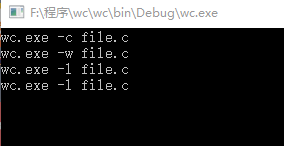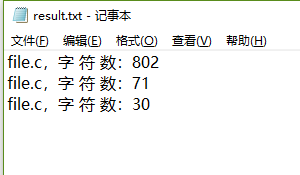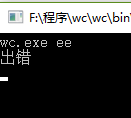LoadFile reader = new LoadFile();
Console.WriteLine(reader.lineNumber);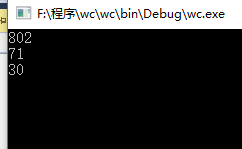string[] type = { "c", "w", "l" };
WriteFile write = new WriteFile();
write.Write("file.c", type); //传入指令和文件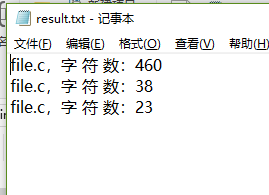posted @ 2018-09-24 21:52  OCAPP  阅读(215)  评论(0编辑  收藏  举报